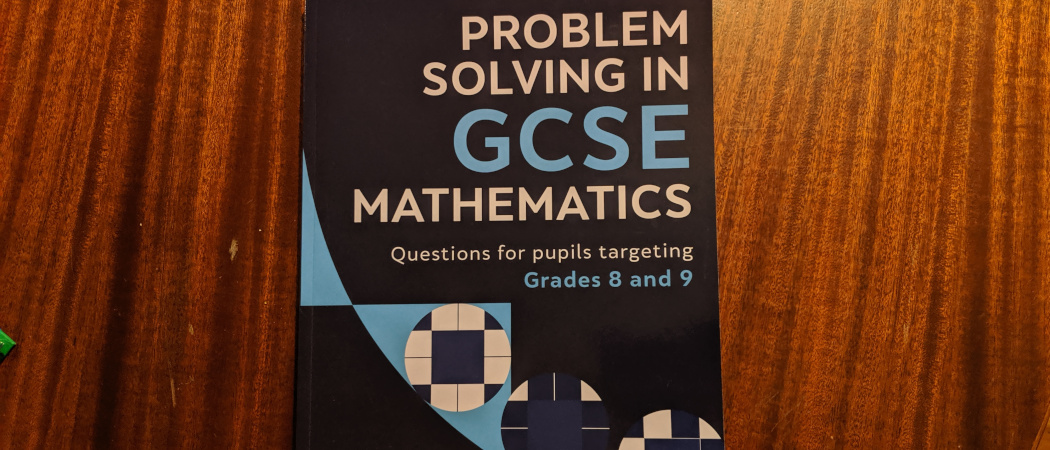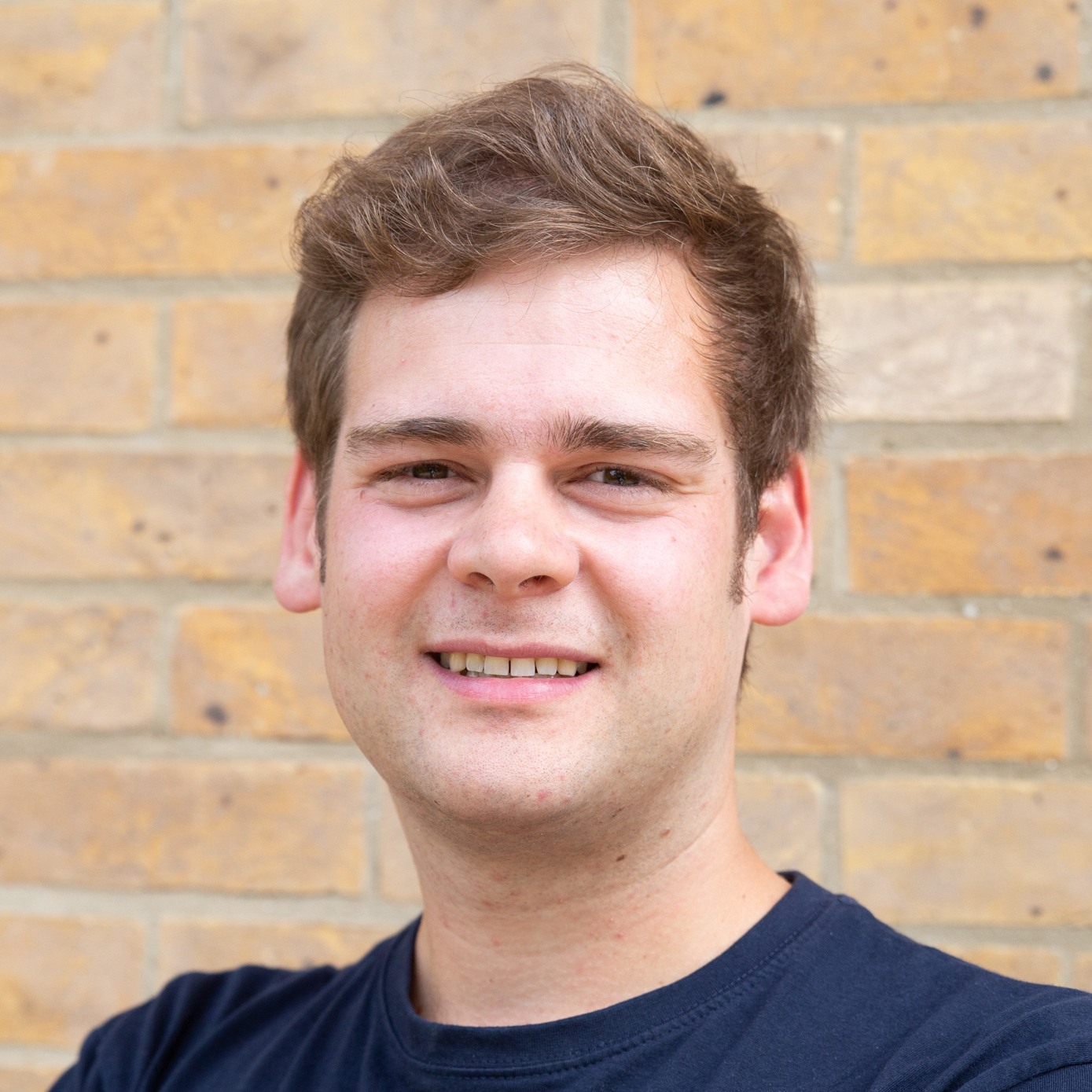# Review of Problem Solving in GCSE Mathematics

We have a go at the puzzles in Daniel Griller’s new bookIn late 2018, Daniel Griller‘s second book, Problem solving in GCSE mathematics was released. I was a big fan of his first book, Elastic numbers, so I was keen to have a go at the puzzles in the sequel.

This book, however, is not like Elastic numbers, but it is instead a workbook containing a collection of problems aimed at GCSE students targeting the top grades. If I had bought this book expecting Elastic numbers 2, I would have been very disappointed. Luckily, the title of this book—Problem solving in GCSE mathematics—tells you exactly what to expect so I was not disappointed.

Problem solving in GCSE mathematics contains 75 questions aimed at students at GCSE level, and is written like a workbook, giving space for students to answer each question, and contains worked solutions for each question. As you might expect from a puzzle writer like Daniel Griller, the questions often present unfamiliar and unusual situations in which familiar bits of GCSE maths can be used. It was questions like these that I found the most enjoyable to solve. Here are a couple of my favourites.

### 12

Alice is thinking of a positive integer N. The lowest common multiple of N and 45 is 90. Find all possible values of N.

### 65

Solve
$$\sqrt{\frac{4x}{15}}+2=\sqrt{\frac{9x}{10}}$$

For the second puzzle in particular, it took me a while to realise that I even knew how to solve it. The book contains many puzzles like this that will get even the highest achieving GCSE students to think about what they know in a different way.

If you are a GCSE student looking to achieve a top grade, or a teacher or parent looking for questions students like this, I would highly recommend this book. If you’re looking for some fun puzzles to do on a Sunday afternoon, this book isn’t really aimed at you: find a student to buy this for and buy Elastic numbers for yourself.Matthew Scroggs is a postdoctoral researcher in the Department of Engineering at the University of Cambridge working on finite and boundary element methods. His website, mscroggs.co.uk, is full of maths.
@mscroggs    mscroggs.co.uk    + More articles by Matthew